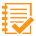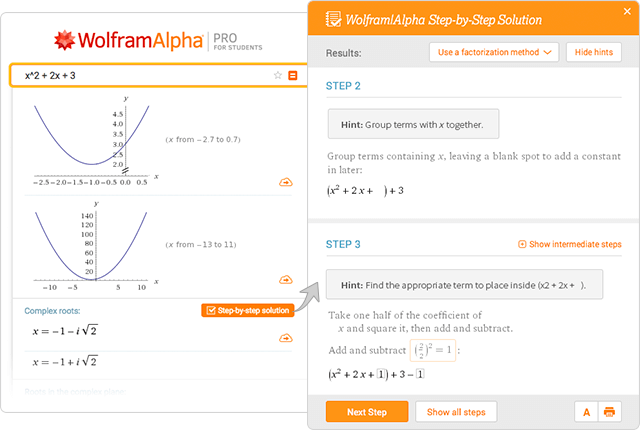Pro

Examples for

#Step-by-Step Solutions

Solutions for over 60 topics within math, chemistry and physics.Arithmetic

More examples
Statistics

#### Perform basic statistical analysis on a set of data, seeing the steps along the way:

More examples
Discrete Mathematics

More examples
Chemistry

More examples
Algebra

More examples
Calculus

More examples
Linear Algebra

More examples

### Physics Formulas

Geometry

#### Determine the equation of a line with certain properties using a selected form:

More examples
Differential Equations

More examples
Proofs

More examples